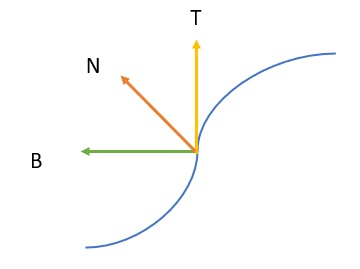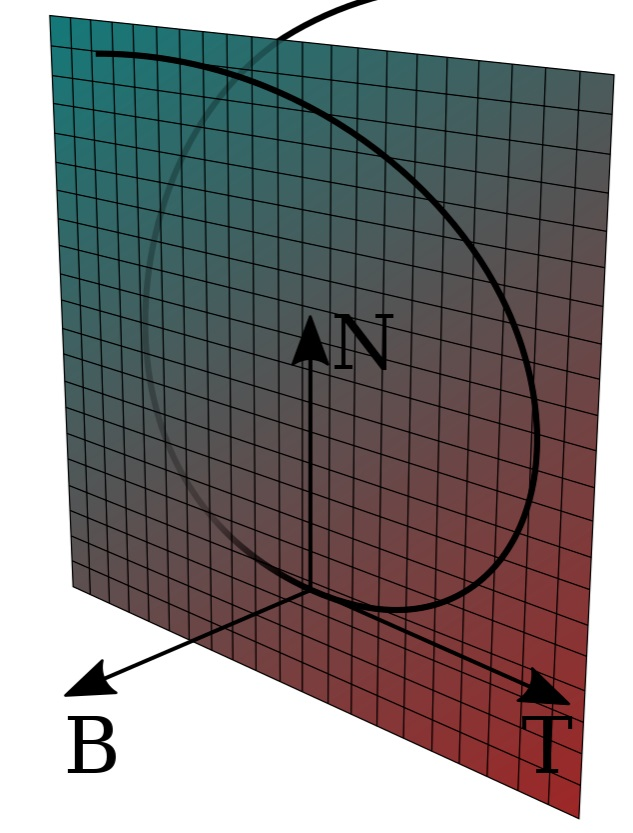# Frenet Frame: Simple Definition

## What is a Frenet Frame?

The Frenet frame (also called the moving trihedron or Frenet trihedron) along a curve is a moving (right-handed) coordinate system determined by the tangent line and curvature. The frame, which locally describes one point on a curve, changes orientation along the length of the curve.Every point on the curve can be described by three natural vectors.

## Formal Definition of Frenet Frame

More formally, the Frenet frame of a curve at a point is a triplet of three mutually orthogonal unit vectors {T, N, B}. In three-dimensions, the Frenet frame consists of :

• The unit tangent vector T, which is the unit vector in the direction of what is being modeled (like velocity),
• The unit normal N: the direction where the curve is turning. We can get the normal by taking the derivative of the tangent then dividing by its length. You can think of the normal as being the place the curve sits in .
• The unit binormal B = T x N, which is the cross product of the unit tangent and unit normal.A space curve; the vectors T, N and B; and the osculating plane spanned by T and N. Image: Popletibus | Wikimedia Commons.

The tangent and normal unit vectors span a plane called the osculating plane at F(s). In four-dimensions, the Frenet frame contains an additional vector, the trinormal unit vector . While vectors have no origin in space, it’s traditional with Frenet frames to think of the vectors as radiating from the point of interest.

The Frenet frame {T, N, B} is called a discrete Frenet frame (DFF). As well as its importance in studying curves, the DFF has some specific uses, including studying shapes of long molecules like proteins .

## References

Image: Popletibus This W3C-unspecified vector image was created with Inkscape, CC BY-SA 4.0 <, via Wikimedia Commons  Green, P. & Rosenberg, J. (2000). Space Curves, FrenetFrames, and Torsion. Retrieved August 5, 2021 from: https://www.math.umd.edu/~jmr/241/curves2.htm  Kho, G. (2013). CS468 Notes.
 Frenet-Serret formulas. Retrieved August 5, 2021 from: https://ncatlab.org/nlab/show/Frenet-Serret+formulas
 u, Y. (2013). Discrete Frenet Frame with Application to Structural Biology and Kinematics. Retrieved August 5, 2021 from: https://diginole.lib.fsu.edu/islandora/object/fsu%3A183801

CITE THIS AS:
Stephanie Glen. "Frenet Frame: Simple Definition" From StatisticsHowTo.com: Elementary Statistics for the rest of us! https://www.statisticshowto.com/frenet-frame/